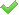# Equation of circle 2

Find the equation of a circle which touches the axis of y at a distance 4 from the origin and cuts off an intercept of length 6 on the axis x.

Result

f = (Correct answer is: )#### Solution:Leave us a comment of example and its solution (i.e. if it is still somewhat unclear...):

Showing 0 comments:Be the first to comment!#### To solve this example are needed these knowledge from mathematics:

For Basic calculations in analytic geometry is helpful line slope calculator. From coordinates of two points in the plane it calculate slope, normal and parametric line equation(s), slope, directional angle, direction vector, the length of segment, intersections the coordinate axes etc. Looking for help with calculating roots of a quadratic equation? Do you have a linear equation or system of equations and looking for its solution? Or do you have quadratic equation? Pythagorean theorem is the base for the right triangle calculator. See also our trigonometric triangle calculator.

## Next similar examples:

1. Equation of circlefind an equation of the circle with indicated properties: a. center at (-3,5), diameter 20. b. center at origin and diameter 16.
2. Circle - AGFind the coordinates of circle and its diameter if its equation is: ?
3. Find parametersFind parameters of the circle in the plane - coordinates of center and radius: ?
4. RT and circlesSolve right triangle if the radius of inscribed circle is r=9 and radius of circumscribed circle is R=23.
5. On lineOn line p: x = 4 + t, y = 3 + 2t, t is R, find point C, which has the same distance from points A [1,2] and B [-1,0].
6. Evaluation of expressionsIf a2-3a+1=0, find (i)a2+1/a2 (ii) a3+1/a3
7. Quadratic equationFind the roots of the quadratic equation: 3x2-4x + (-4) = 0.
8. CalculateCalculate the length of a side of the equilateral triangle with an area of 50cm2.
9. Square root 2If the square root of 3m2 +22 and -x = 0, and x=7, what is m?
10. Right angled triangleHypotenuse of a right triangle is 17 cm long. When we decrease length of legs by 3 cm then decrease its hypotenuse by 4 cm. Determine the size of legs.
11. EquationEquation ? has one root x1 = 8. Determine the coefficient b and the second root x2.
12. RootsDetermine the quadratic equation absolute coefficient q, that the equation has a real double root and the root x calculate: ?
13. CathetiThe hypotenuse of a right triangle is 41 and the sum of legs is 49. Calculate the length of its legs.
14. Solve 3Solve quadratic equation: (6n+1) (4n-1) = 3n2
15. DiscriminantDetermine the discriminant of the equation: ?
16. Circle annulusThere are 2 concentric circles in the figure. Chord of larger circle 10 cm long is tangent to the smaller circle. What are does annulus have?
17. Theorem proveWe want to prove the sentense: If the natural number n is divisible by six, then n is divisible by three. From what assumption we started?